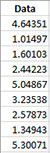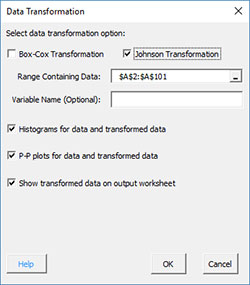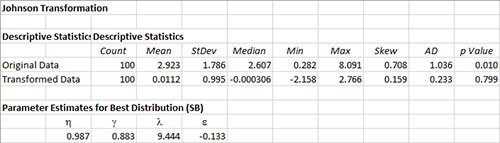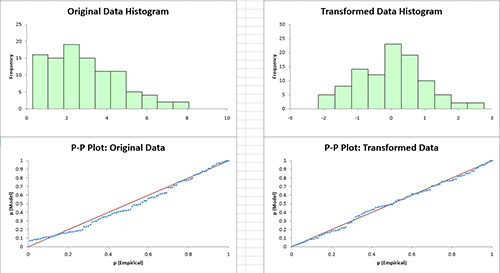# Johnson Transformation HelpThis page shows how to perform the Johnson Transformation using SPC for Excel.  The data can be downloaded at this link.

### Running the Johnson Transformation

1. Enter data into a worksheet as shown below (only a portion of the 100 data points shown).2. Select the data on the worksheet to be included in the analysis.  This is the shaded area shown below.  You can use “Select Cells” in the “Utilities” panel of the SPC for Excel ribbon to quickly select the cells.3. Select “Data Transformation” on the “Distributions” panel on the SPC for Excel ribbon.

4. The input form below is displayed.  Select the “Johnson Transformation” option.• Range Containing Data: select the range containing the data; the default is the range selected on the worksheet
• Variable Name (Optional): enter the name the variable (note: this will populate with the first cell in the selected range if it contains text)
• Histograms for data and transformed data: this options creates histograms for the original data and the transformed data so you can visually see if the transformed data appears normal; default is Yes.
• P-P plots for data and transformed data: this options creates the P-P plot for the original data and the transformed data; if the data falls along a straight line, the data are normally distributed; default is Yes
• The P-P plot plots the empirical CDF against the theoretical CDF value.
• Show transformed data on output worksheet: this option prints the original data on the worksheet along with the transformed data
• Select OK to run the Johnson  transformation
• Select Cancel to exit the program

### Johnson Transformation Output

Two sheets are added to the workbook.  Both are named  with a number following the name reflecting the number of times the Johnson transformation has been run in the workbook.

JT Plot

This sheet contains the Johnson transformation and includes the best distribution (SB in this example), p value, the z value and the transformation equation.  All three transformations are plotted.JT Output

The top part of this worksheet contains the descriptive statistics for original and transformed data.   The coefficients from the Johnson transformation are also shown.The middle part of the worksheet contains the histograms and P-P plots (if those options were selected).The bottom part of the worksheet contains the transformed data if that options was selected.